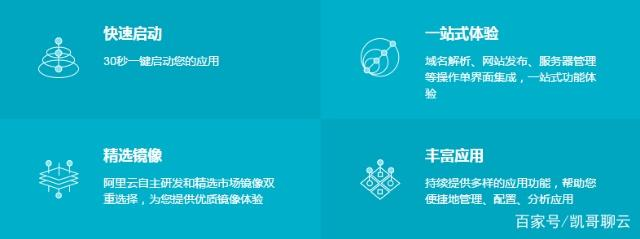+关注继续查看

# 前言

1. 就是买了 IaaS 却不会用
2. PaaS、SaaS 的自由度不高
3. ECS 附加功能太多还用不到，价格贵

# 介绍

1. 轻量应用服务器 是 IaaS 产品但是提供定制化应用镜像且有可视化控制台可以操作，不怕新手不会用。轻量应用服务器 在提供
2. 提供定制化应用镜像的同时，用户依旧可以拥有 root 权限，对系统进行个性化操作
3. 精简了一些 ECS 的功能，比如说安骑士。把安全组的操作也做了适当减法更适合用户操作# 优点

## 控制台ECS## 应用镜像## 功能ECS 能就只有空白的系统镜像，任何环境都是需要用户自行安装的，这在一定程度上增加了用户使用的学习成本。而 ECS 更多体现的就是专业性了，虽然复杂但是十分强大的安全组、弹性IP、均衡负载等等。

## 基础运维## 防火墙# 缺点

## 三大金刚的版本问题

Apache Httpd、PHP、MySQL 是会持续更新的，而且它们也均有爆发过大规模严重漏洞的历史，不过目前还没有看到应用镜像中的三大金刚如何升级版本号的姿势。

# 评测

## CPU 性能

System Benchmarks Index Values               BASELINE       RESULT    INDEX
Dhrystone 2 using register variables         116700.0   34281977.0   2937.6
Double-Precision Whetstone                       55.0       2962.7    538.7
Execl Throughput                                 43.0       4860.0   1130.2
File Copy 1024 bufsize 2000 maxblocks          3960.0    1054197.7   2662.1
File Copy 256 bufsize 500 maxblocks            1655.0     283098.8   1710.6
File Copy 4096 bufsize 8000 maxblocks          5800.0    3165160.0   5457.2
Pipe Throughput                               12440.0    1734490.3   1394.3
Pipe-based Context Switching                   4000.0     375732.0    939.3
Process Creation                                126.0      18345.1   1456.0
Shell Scripts (1 concurrent)                     42.4       6747.0   1591.3
Shell Scripts (8 concurrent)                      6.0        921.5   1535.8
System Call Overhead                          15000.0    2939229.7   1959.5
========
System Benchmarks Index Score                                        1649.5

ECS：

System Benchmarks Index Values               BASELINE       RESULT    INDEX
Dhrystone 2 using register variables         116700.0   34582858.9   2963.4
Double-Precision Whetstone                       55.0       2984.8    542.7
Execl Throughput                                 43.0       5118.8   1190.4
File Copy 1024 bufsize 2000 maxblocks          3960.0    1073823.9   2711.7
File Copy 256 bufsize 500 maxblocks            1655.0     284701.5   1720.3
File Copy 4096 bufsize 8000 maxblocks          5800.0    3213933.6   5541.3
Pipe Throughput                               12440.0    1722785.7   1384.9
Pipe-based Context Switching                   4000.0     377999.7    945.0
Process Creation                                126.0      18704.4   1484.5
Shell Scripts (1 concurrent)                     42.4       6852.6   1616.2
Shell Scripts (8 concurrent)                      6.0        937.2   1562.0
System Call Overhead                          15000.0    2979190.8   1986.1
========
System Benchmarks Index Score                                        1673.4

## 磁盘性能

/dev/vda:
Timing cached reads:   21070 MB in  2.00 seconds = 10549.25 MB/sec
Timing buffered disk reads: 270 MB in  3.01 seconds =  89.76 MB/sec

[root@izbp1auqkyqtj4iozs7athz dev]# fio --bs=4k --ioengine=libaio --iodepth=1 --direct=1 --rw=read --time_based --runtime=600 --refill_buffers --norandommap --randrepeat=0 --group_reporting --name=fio-read --size=10G --filename=/dev/vda
fio-2.2.8
Starting 1 process
Jobs: 1 (f=1): [R(1)] [100.0% done] [7200KB/0KB/0KB /s] [1800/0/0 iops] [eta 00m:00s]
fio-read: (groupid=0, jobs=1): err= 0: pid=24361: Sun Sep 10 15:04:27 2017
read : io=4206.7MB, bw=7179.3KB/s, iops=1794, runt=600004msec
slat (usec): min=3, max=165, avg= 6.78, stdev= 2.02
clat (usec): min=2, max=84748, avg=548.56, stdev=2727.93
lat (usec): min=131, max=84755, avg=555.59, stdev=2727.93
clat percentiles (usec):
|  1.00th=[  141],  5.00th=[  241], 10.00th=[  302], 20.00th=[  306],
| 30.00th=[  314], 40.00th=[  322], 50.00th=[  334], 60.00th=[  350],
| 70.00th=[  362], 80.00th=[  382], 90.00th=[  442], 95.00th=[  502],
| 99.00th=[ 1012], 99.50th=, 99.90th=, 99.95th=,
| 99.99th=
bw (KB  /s): min= 4624, max= 7784, per=100.00%, avg=7182.85, stdev=181.15
lat (usec) : 4=0.01%, 250=6.25%, 500=88.69%, 750=3.79%, 1000=0.27%
lat (msec) : 2=0.36%, 4=0.06%, 10=0.03%, 20=0.04%, 50=0.48%
lat (msec) : 100=0.04%
cpu          : usr=0.50%, sys=1.81%, ctx=1076903, majf=1, minf=33
IO depths    : 1=100.0%, 2=0.0%, 4=0.0%, 8=0.0%, 16=0.0%, 32=0.0%, >=64=0.0%
submit    : 0=0.0%, 4=100.0%, 8=0.0%, 16=0.0%, 32=0.0%, 64=0.0%, >=64=0.0%
complete  : 0=0.0%, 4=100.0%, 8=0.0%, 16=0.0%, 32=0.0%, 64=0.0%, >=64=0.0%
issued    : total=r=1076894/w=0/d=0, short=r=0/w=0/d=0, drop=r=0/w=0/d=0
latency   : target=0, window=0, percentile=100.00%, depth=1

Run status group 0 (all jobs):
READ: io=4206.7MB, aggrb=7179KB/s, minb=7179KB/s, maxb=7179KB/s, mint=600004msec, maxt=600004msec

vda: ios=1078167/690, merge=0/399, ticks=598805/4230, in_queue=602927, util=98.01%


ECS：

/dev/vdb:
Timing cached reads:   19778 MB in  2.00 seconds = 9901.01 MB/sec
Timing buffered disk reads: 270 MB in  3.02 seconds =  89.54 MB/sec

[root@iZbp1258gr0v9v184jdqqkZ dev]# fio --bs=4k --ioengine=libaio --iodepth=1 --direct=1 --rw=read --time_based --runtime=600 --refill_buffers --norandommap --randrepeat=0 --group_reporting --name=fio-read --size=10G --filename=/dev/vdb
fio-2.2.8
Starting 1 process
Jobs: 1 (f=1): [R(1)] [100.0% done] [6940KB/0KB/0KB /s] [1735/0/0 iops] [eta 00m:00s]
fio-read: (groupid=0, jobs=1): err= 0: pid=9552: Sun Sep 10 15:23:44 2017
read : io=4218.9MB, bw=7200.2KB/s, iops=1800, runt=600001msec
slat (usec): min=3, max=344, avg= 6.97, stdev= 2.32
clat (usec): min=1, max=85711, avg=546.79, stdev=4741.30
lat (usec): min=145, max=85717, avg=553.99, stdev=4741.28
clat percentiles (usec):
|  1.00th=[  147],  5.00th=[  149], 10.00th=[  151], 20.00th=[  153],
| 30.00th=[  155], 40.00th=[  159], 50.00th=[  167], 60.00th=[  199],
| 70.00th=[  229], 80.00th=[  241], 90.00th=[  249], 95.00th=[  270],
| 99.00th=[  374], 99.50th=, 99.90th=, 99.95th=,
| 99.99th=
bw (KB  /s): min= 6380, max= 7624, per=100.00%, avg=7202.00, stdev=111.69
lat (usec) : 2=0.01%, 50=0.01%, 250=90.56%, 500=8.76%, 750=0.06%
lat (usec) : 1000=0.02%
lat (msec) : 2=0.02%, 4=0.01%, 10=0.01%, 20=0.01%, 50=0.01%
lat (msec) : 100=0.55%
cpu          : usr=0.50%, sys=1.98%, ctx=1080029, majf=0, minf=34
IO depths    : 1=100.0%, 2=0.0%, 4=0.0%, 8=0.0%, 16=0.0%, 32=0.0%, >=64=0.0%
submit    : 0=0.0%, 4=100.0%, 8=0.0%, 16=0.0%, 32=0.0%, 64=0.0%, >=64=0.0%
complete  : 0=0.0%, 4=100.0%, 8=0.0%, 16=0.0%, 32=0.0%, 64=0.0%, >=64=0.0%
issued    : total=r=1080027/w=0/d=0, short=r=0/w=0/d=0, drop=r=0/w=0/d=0
latency   : target=0, window=0, percentile=100.00%, depth=1

Run status group 0 (all jobs):
READ: io=4218.9MB, aggrb=7200KB/s, minb=7200KB/s, maxb=7200KB/s, mint=600001msec, maxt=600001msec

vdb: ios=1079640/0, merge=0/0, ticks=589523/0, in_queue=589382, util=98.27%50 01480 04281 0675 029292 0【云计算的1024种玩法】ECS和轻量应用服务器的远程控制入门

6624 019394 013635 015336 0144 0+关注

107

5037

JS零基础入门教程（上册）